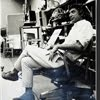## Eugene Ray SDSU 1970'sEugene Ray 1970's. photo credit: Tom Davis

## Monday, July 15, 2013

### PLANTATION PROTOTYPE FOR LONGER LIFE+CASTLE AS ARCHTYPE+
>ancient castles provided dynamic<
+MULTI-VALENT+
>support for extended families<
^^^^^^^^^^^^^^^^^^^^^^

+HEALING SYNERGETICS+
> concept of a modern plantation for the<
>combined Ray/de Bresse families<
^^^^^^^^^^^^^^^^^^^^^^^^^
+MODERN CASTLE+
>as inspired by Gruy/Bresse ancestors<
>with Savoy French Swiss blood<
^^^^^^^^^^^^^^^^^^^^^^^^^
+THE COUNTS OF GRUYERE+
>Eugene Ray Architect<
>Gruyere Castle<
^^^^^^^^^^^
(>for several years I have sent this<
>nightly blog to my friends at<
+GRUYERE CASTLE+)
^^^^^^^^^^^^^^^

+TONIGHT'S PHOTOS+
>the professor's most honored role<
>is as a visionary architect with<
>a devotion to prototypes<
^^^^^^^^^^^^^^^^^^^

#1).                    +HOLOGRAPHIC+
>vision to Gruy/Bresse/Savoy blood<
>is drawn from Genetic Memory<
+WWW AVAILABLE+
>galvanized by this Gruy book<
^^^^^^^^^^^^^^^^^^
(>note: I have told the story of the<
>miracle of finding this book<)
^^^^^^^^^^^^^^^^^^^^^

#2).           +PLANTATION PROTOTYPE+
>for longer living was designed to have<
>three generations of extended family<
(+RAY=BRES+)
>nourished by the plant kingdom<
>(read "the secret life of plants")<
^^^^^^^^^^^^^^^^^^^^^^^^^

#3).         +PLANTATION PROTOTYPE+
>for longer living is wind and solar energy<
>supported with biotronic energy in<
+CONICAL=SPHERICAL+
>enclosed separate family suites<
^^^^^^^^^^^^^^^^^^^^^^^
#4).            +"DESERT INCIDENTS"+
>my original vision for an Arcadian type<
>concepts also was castle inspired<
^^^^^^^^^^^^^^^^^^^^^^^^^^
#5).               +GRUYERE CASTLE+
>our ancient family archetype where the<
+COUNTS OF GRUYERE+
>lived for over five hundred years and<
>inspired much holographic vision<
^^^^^^^^^^^^^^^^^^^^^^^^

+EXTENDED FAMILIES+
>in one complex prototype share the<
>major living, dining, kitchen, work<
>spaces providing many virtues<
^^^^^^^^^^^^^^^^^^^^^^
+FAMILY INTERACTION+
>very special<
+WITH ECONOMY+
^^^^^^^^^^^^^
Eugene Ray, mfa, Architect
Professor Emeritus, sdsu
^^^^^^^^^^^^^^^^^^